# Multiplier theory

Given a Fourier series on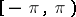,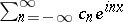say, and a (doubly infinite) sequence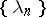, one may form a new Fourier series,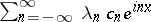. The sequenceis called a Fourier multiplier. The principal problem about Fourier multipliers is to determine conditions onwhich guarantee that, when the old Fourier series corresponds to an element of some space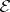of functions or generalized functions (cf. Generalized function) on, then the new series corresponds to an element of some other given space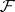of functions or generalized functions on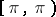. Typically,andare Lebesgue spaces, Sobolev spaces or similar function spaces (cf. Lebesgue space; Sobolev space). Particular cases of the problem were first solved by W.H. Young (1913), H. Steinhaus (1915) and S. Sidon (1921), the most significant of these solutions being thatis a multiplier from the space of integrable functions to itself or from the space of continuous functions to itself if and only ifis a Fourier–Stieltjes series. Equivalently, one can seek to characterize generalized functionsonwith the property that, if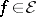, then the convolution product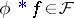; the corresponding Fourier multiplier is the sequence of Fourier coefficients of.
The most important results on Fourier multipliers are connected with the theories of singular integral operators and pseudo-differential operators (cf. Pseudo-differential operator). The general style of these is exemplified by the theorem that a bounded functiononwhose total variation (cf. Variation of a function) on each dyadic intervalis bounded is a Fourier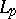-multiplier (i.e. the associated operator mapsinto itself) if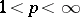. Perhaps the other most important result in the field is C. Fefferman's theorem that in, where, the characteristic function of the unit ball is a Fourier-multiplier only if.